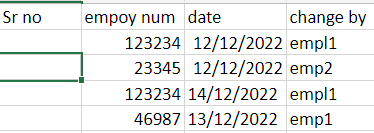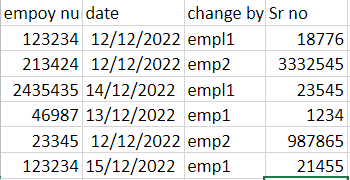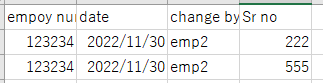# Linq Query for comparing datatable

Hi,

I have 2 datatables .DT1 and DT2I have to check if clms B,C,D from DT1 matches with clms A,B,C of DT2 and if it mathes i have to write srno value from DT2 to srno clm in DT1 correspondingly. I dont want to use excel for this. Is there any linq query for this?

Hi,

``````dict = dt2.AsEnumerable.ToDictionary(Function(r) Tuple.Create(r("empoy num").ToString,r("date").ToString,r("change by").ToString),Function(r) r("Sr no").ToString)
``````

Then

``````dt1 = dt1.AsEnumerable.Select(Function(r) dt1.Clone.LoadDataRow({if(dict.ContainsKey(Tuple.Create(r("empoy num").ToString,r("date").ToString,r("change by").ToString)),CObj(dict(Tuple.Create(r("empoy num").ToString,r("date").ToString,r("change by").ToString))),CObj(""))}.Concat(r.ItemArray.Skip(1)).ToArray,False)).CopyToDataTable
``````

Sample20221129-5.zip (10.6 KB)

Regards,

Can you explain the query?

Hi,

I tried this code but im getting an error like “An item with the same key has already been added”

This is a datatbase operation called a join. You can use Join Data Tables for this.

HI,

It’s because there are duplicated set of “empoy num”,“date” and “change by”

If it’s completely duplicated record, we can easily have them one record.
If they have different Sr no as the following, we need to rule which should be selected.For example, the following sample selects first record if duplicate record.
Can you try this?

``````dict = dt2.AsEnumerable.GroupBy(Function(r) Tuple.Create(r("empoy num").ToString,r("date").ToString,r("change by").ToString)).ToDictionary(Function(g) g.key,Function(g) g.First().Item("Sr no").ToString)
``````

Sample20221129-5V2.zip (10.6 KB)

Regards,

Thanks,this worked

1 Like

This topic was automatically closed 3 days after the last reply. New replies are no longer allowed.